# Difference between revisions of "Second derivative"

Jump to: navigation, search

## Definition at a point

### Definition in terms of first derivative

The second derivative of a function$f$ at a point$x_0$, denoted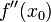$\! f''(x_0)$, is defined as the derivative at the point$x_0$ of the function defined as the derivative$f'$

Note that the first differentiation operation must be performed, not just at the point, but at all points near it, so that we have a function for the first derivative around the point, which we can then differentiate to calculate the second derivative at the point. It is not good enough to calculate the first derivative only at the particular point (i.e., to calculate only$f'(x_0)$) and then proceed to differentiate that; we need the value of the first derivative at nearby points too.

### Definition as a limit expression

The second derivative of a function$f$ at a point$x_0$, denoted$\! f''(x_0)$, is defined as follows: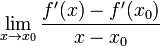$\! \lim_{x \to x_0} \frac{f'(x) - f'(x_0)}{x - x_0}$

More explicitly, this can be written as:$\! \lim_{x \to x_0} \frac{1}{x - x_0}\left[\lim_{x_1 \to x} \frac{f(x_1) - f(x)}{x_1 - x} - \lim_{x_2 \to x_0} \frac{f(x_2) - f(x_0)}{x_2 - x_0}\right]$

## Definition as a function

The second derivative of a function at a point is defined as the derivative of the derivative of the function. For a function$f$, the second derivative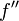$f''$ is defined as:$\! f'' := (f')'$

## Leibniz notation for second derivative

Suppose$f$ is a function, and$x,y$ are variables related by$y := f(x)$. Here,$x$ is an independent variable and$y$ is the dependent variable (with the dependency being described by the function$f$). We then define: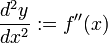$\frac{d^2y}{dx^2} := f''(x)$

This can also be written as:$\frac{d^2}{dx^2}[f(x)]$

In particular,$d^2y/dx^2$ is a function of$x$. Its value at$x = x_0$ is defined as$f''(x_0)$ and is denoted as follows: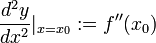$\! \frac{d^2y}{dx^2} |_{x = x_0} := f''(x_0)$

## Significance

### Significance of sign on intervals

Loose statement Precise statements
concave up function means derivative is increasing means second derivative is positive positive second derivative implies concave up
Fill this in later
concave down function means derivative is decreasing means second derivative is negative negative second derivative implies concave down
linear function (or constant function) means derivative is constant means second derivative is zero

### Significance of sign at points

A point of inflection is a point of geometric significance on the graph (where the function changes its sense of concavity), and corresponds to a point in the domain of the function where the second derivative changes sign. A point of inflection must correspond to a point of (strict) local maximum or minimum for the first derivative.

Method for constructing new functions from old In symbols Derivative in terms of old functions and their first, second derivatives Proof
pointwise sum$f + g$ is the function$x \mapsto f(x) + g(x)$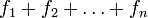$f_1 + f_2 + \dots + f_n$ is the function$x \mapsto f_1(x) + f_2(x) + \dots + f_n(x)$
Sum of the second derivatives of the functions being added (the second derivative of the sum is the sum of the second derivatives)$\! f'' + g''$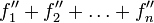$\! f_1'' + f_2'' + \dots + f_n''$
repeated differentiation is linear
pointwise difference$f - g$ is the function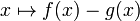$x \mapsto f(x) - g(x)$ Difference of the second derivatives, i.e.,$f'' - g''$ repeated differentiation is linear
scalar multiple by a constant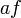$af$ is the function$x \mapsto af(x)$ where$a$ is a real number$x \mapsto af''(x)$ repeated differentiation is linear
pointwise product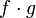$f \cdot g$ (sometimes denoted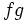$fg$) is the function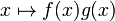$x \mapsto f(x)g(x)$$f_1 \cdot f_2 \cdot \dots f_n$ (sometimes denoted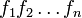$f_1f_2\dots f_n$ is the function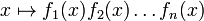$x \mapsto f_1(x)f_2(x) \dots f_n(x)$
For two functions,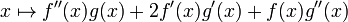$x \mapsto f''(x)g(x) + 2f'(x)g'(x) + f(x)g''(x)$
For multiple functions, more complicated
product rule for higher derivatives
pointwise quotient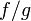$f/g$ is the function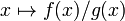$x \mapsto f(x)/g(x)$ Fill this in later quotient rule for higher derivatives
composite of two functions$f \circ g$ is the function$x \mapsto f(g(x))$$x \mapsto f''(g(x))(g'(x))^2 + f'(g(x))g''(x)$ chain rule for higher derivatives
inverse function of a one-one function$f^{-1}$ sends$x$ to the unique$y$ such that$f(y) = x$$\! \frac{-f''(f^{-1}(x))}{(f'(f^{-1}(x)))^3}$ higher derivatives of inverse function
piecewise definition Fill this in later Fill this in later differentiation rule for piecewise definition by interval